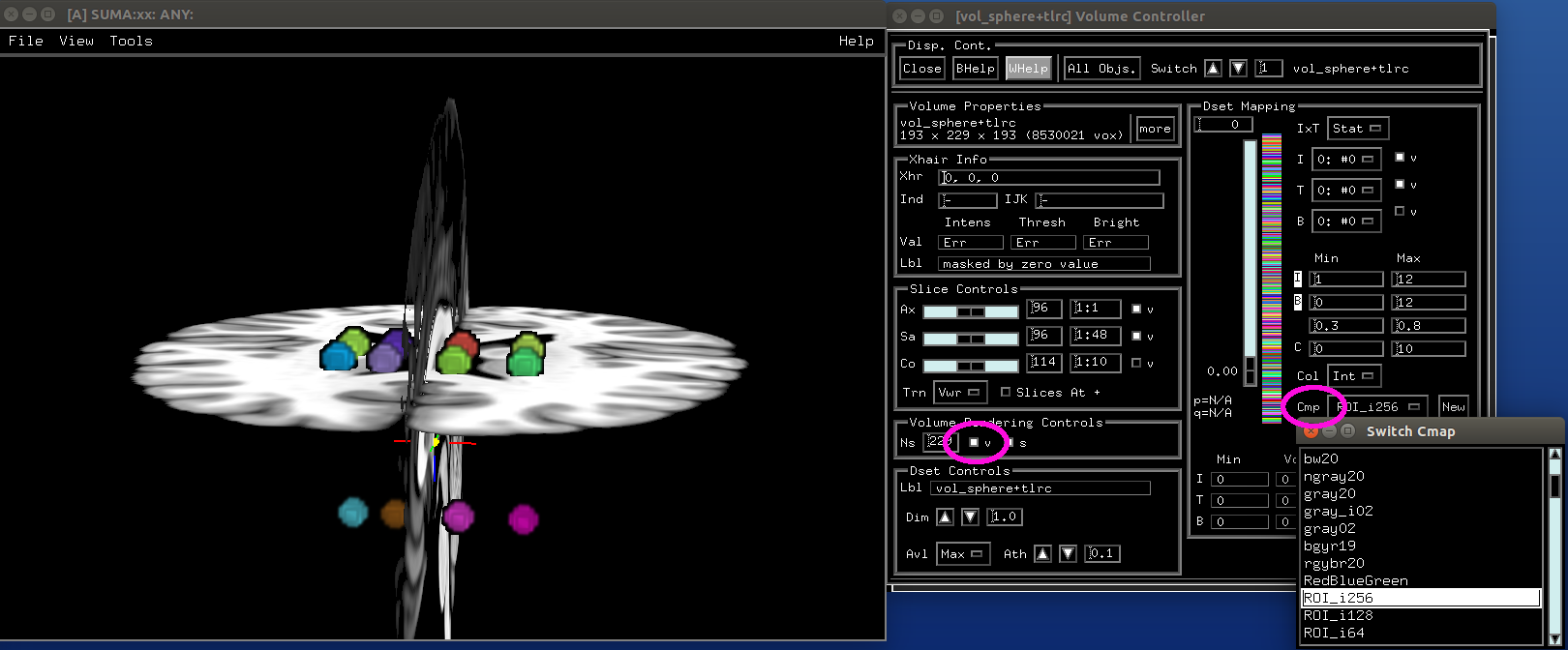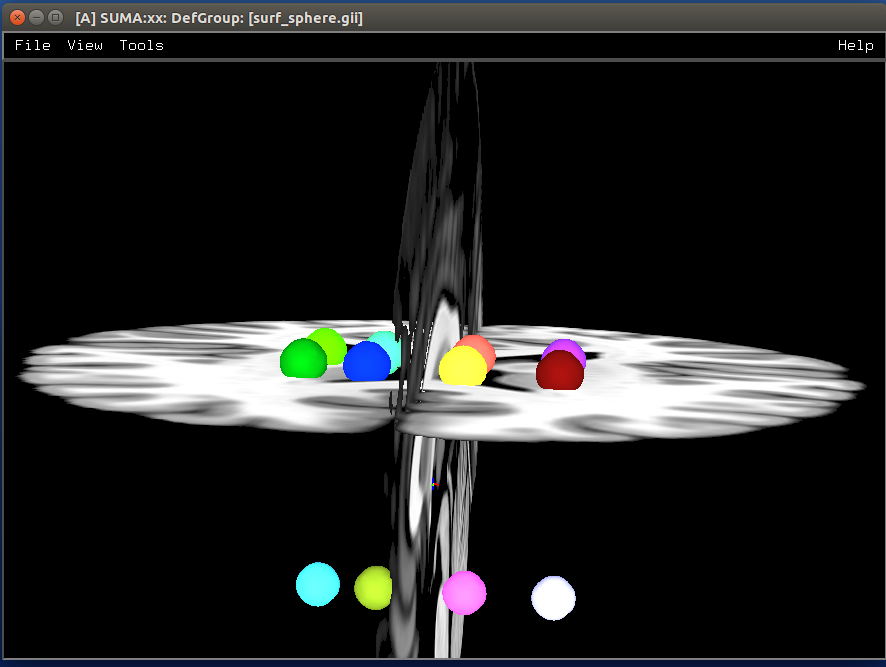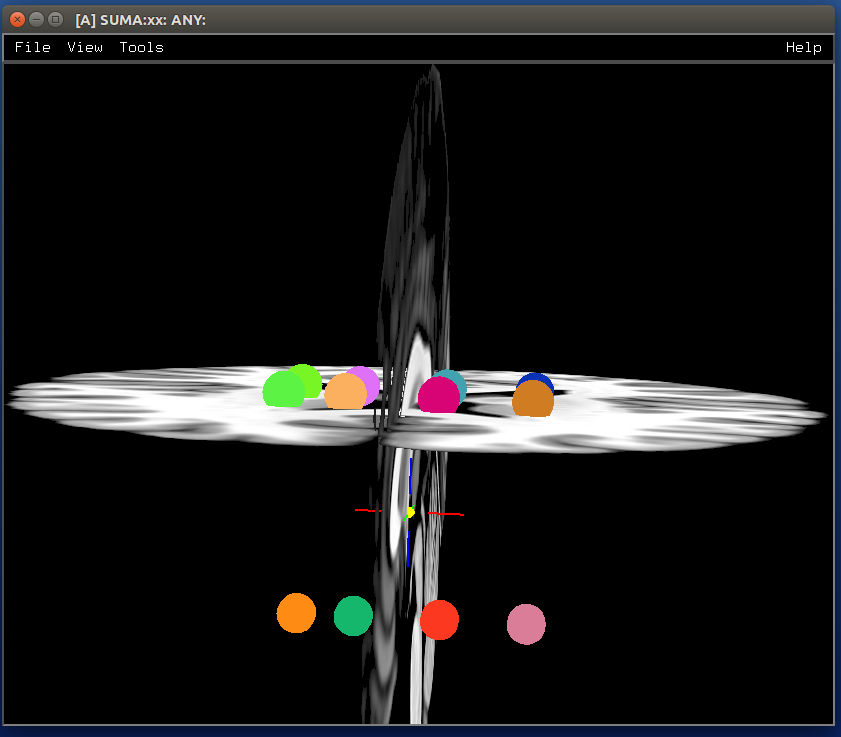# 12.14.2. Manifold ways to make+view spheres in SUMA¶

## Description¶

This tutorial starts with:

• a list of coordinates representing the center of spheres

• a dset that provides a background/grid (here, MNI space)

and shows how to:

• make+view spheres various ways in SUMA.

## Survey of approaches¶

Spherical objects can be rendered in 4 general ways in suma (plus some extra variants, noted in the script/examples).

1. NIDO: NeuroImaging Displayable Objects

There’s a demo for this in the distribution (@DO_examples), and I created a fun script for April Fool’s day that shows one sphere. These objects aren’t clickable in suma; they are only for display. The @DO_examples script needs a little modification for recent Mac OS to keep the screen controller open first. The April Fool’s Day posting here was for fun, but it does have a serious use - like yours.

2. Manufactured spherical surfaces (2 main kinds)

These are all clickable. That means clicking on a sphere identifies the node in some way. The coordinate can also be shared with the afni GUI if the two programs are talking to each other. Here again, you can do this multiple ways:

1. CreateIcosahedron - create an icosahedron or project to a sphere. The RAVE software (R Analysis and Visualization of intracranial EEG Data) by the Beauchamp lab uses this approach:

2. From volumetric datasets of spheres or real electrode geometry, as seen in CT images of ECOG. The ALICE software creates “spheres” based on the CT images or positions marked. The spheres are all made in the volume at the resolution of the dataset, so the conversion to a surface by IsoSurface is a little rough. These can be indexed sequentially as a “merged” surface of multiple surfaces, and colored by color maps or color planes in suma. For the ALICE software, all the electrodes, usually about 100, are colored separately. As each is selected, we turn off or dim the color by manipulating the color map where the sphere index is loaded (1-100) and then colored initially with a niml dataset, then colored with a color map suitable for ROI indices. The color planes offer an alternative way to color a surface; each node index can be colored separately.

ALICE: A tool for automatic localization of intra-cranial electrodes for clinical and high-density grids Mariana P. Branco, et al., 2017. Journal of Neuroscience Methods 301 DOI: 10.1016/j.jneumeth.2017.10.022.

3. Direct volumetric display

This is the least sophisticated way, but it is also the easiest. Make spheres in a volume with 3dUndump, each with a separate value for the sphere. There will be 4 columns in the input to 3dUndump for the xyz coordinates and the value to assign to the sphere. Show in suma with suma -vol myspheres+orig. Render with suma or even with afni’s Render plugin.

4. Graph nodes object

SUMA can also display “graphs”: essentially, nodes connected by edges. The nodes themselves are spheres, and thus they also count as a method for displaying those geometrical forms. The “bonus” aspect of this approach is that the edges can provide additional information/relationships among the spherical ROIs, such as structural or functional properties (connections, correlation, FA value, etc.). This has been useful in displaying atlas connections, for example in the D99 macaque atlas work (Reveley et al., 2017).

“Three-dimensional digital template atlas of the macaque brain” Reveley, Gruslys, Ye, Samaha, Glen, Russ, Saad, Seth, Leopold, Saleem, 2017. Cerebral Cortex, 27(9):4463-4477, https://doi.org/10.1093/cercor/bhw248”

## Example Setup¶

You can download the individual script and supplementary files for running this demo here:

The pieces of this demo are also shown one-by-one below, so one can read along with them.

Files provided here include:

• sphere_coords.1D

a list of (x, y, z) coordinates that represent the centers of some spheres.

• ROI_i256.1D.cmap

a “colormap”, here a list of (R, G, B) color specifications. This one was made by hitting the “w” over the ROI_i256 colorbar in the SUMA object controller.

• demo_suma_spheres.tcsh

a script file to run all the commands, making spheres and displaying suma commands to view them (can be copy+pasted into terminal). Various warnings abound when running this script, but those are ignorable. The script can be run with:

tcsh demo_suma_spheres.tcsh


## Running the examples¶

### Setup¶

This first step is just to create some additional files that we will need (the set of ROIs) and want (a skull-stripped anatomical) for the demo.

- show code y/n -
#!/bin/tcsh

# script:  demo_suma_spheres.tcsh
# ver   :  1.0
# date  :  Feb 7, 2019
# author:  DR Glen (NIMH, SSCC)
#
# Several methods for showing spheres in SUMA.
#
# ======================================================================

# -------------------- Defining initial files --------------------------

# Start with a list of center coords and a chosen dset space
set sphere_coords = sphere_coords.1D
set templ         = MNI152_T1_2009c+tlrc
set templ_full    = @FindAfniDsetPath "${templ}"/${templ}


### Make volumes and display directly in SUMA¶

- show code y/n -
# -------------------- Preliminary steps ------------------------------

# Count how many spheres
set nsphere = 1dcat ${sphere_coords} | wc -l set lastrow = ccalc -int -expr "${nsphere}-1"

# Make some labels for each sphere.  Here, just a number (~counting or
# indexing).
count -digits 3 -col 1 ${nsphere} > vol_sphere_labels.1D # Combine the labels with the coordinates 1dcat${sphere_coords} vol_sphere_labels.1D > vol_sphere_coords_labeled.1D

# -------------------- Make volumes -----------------------------------

# Create spheres in a volume (from which we will make surfaces)
3dUndump                                  \
-master ${templ_full} \ -srad 3.5 \ -prefix vol_sphere \ -datum byte \ -overwrite \ -xyz \ -orient RAI \ vol_sphere_coords_labeled.1D # This volume can be shown directly in suma or in the afni GUI and in # the afni GUI's render plugin cat << EOF ++ ----------------------------------------------------------------- 0) View the volume (as slices) in SUMA with: suma -vol vol_sphere+tlrc ... where you might want to hit ctrl+s to open the object controller, and then select the square by 'v' in 'Volume Rendering Controls' to view the spheres volumetrically. Also, you can include the reference volume for background purposes, via: suma -vol vol_sphere+tlrc \ -vol${templ_full}

++ -----------------------------------------------------------------

EOF


sphere_00_vol.png### Make isosurfaces of the volumetric ROIs¶

- show code y/n -
# ----------------------- Surfaceize the volumes ------------------------

# Make surfaces (removing any ones that might exist from a previous
# run)
IsoSurface                               \
-overwrite                           \
-isorois                             \
-mergerois+dsets                     \
-o surf_sphere.gii                   \
-input vol_sphere+tlrc               \
-Tsmooth 0.3 60

cat << EOF

++ -----------------------------------------------------------------

1) View the surfaces in SUMA

suma -onestate -i surf_sphere*.gii

or

suma -onestate -i surf_sphere*.gii \
-vol ${templ_full} ++ ----------------------------------------------------------------- EOF  sphere_01_surf.png### Make icosahedrons¶ - show code y/n - # ------------------- Icosahedrons ---------------------------- # Pre-clean (e.g., if re-running demo). set ccc = \ls ico_* if ( "$#ccc" != "0" ) then
\rm ${ccc} endif # Make icosahedrons foreach icoi ( count -digits 3 1${nsphere} )
set xyz = head -$icoi${sphere_coords} | tail -1

CreateIcosahedron                       \
-overwrite                          \
-tosphere                           \
-ctr $xyz \ -prefix ico_${icoi}.gii             \
-ld 20

if ( "$icoi" == "001" ) then SurfaceMetrics -i ico_${icoi}.gii -coords
endif

1deval                                  \
-a ico_001.gii.coord.1D.dset        \
-expr "$icoi" > ico_${icoi}.1D
end

# Combine the icosahedrons together in one surface file.
ConvertSurface                              \
-overwrite                              \
-onestate                               \
-anatomical                             \
-merge_surfs                            \
-i ico_0??.gii                          \
-o ico_combined.gii

cat ico_???.1D > ico_combined.1D

# The niml dset should be a little easier to work with, because
ConvertDset                                  \
-overwrite                               \
-i ico_combined.1D                       \
-o ico_combined.niml.dset

#\rm -f ico_*.spec

cat << EOF

++ -----------------------------------------------------------------

2) View the icosahedrons in SUMA

suma -i ico_combined.gii

or

suma -i ico_combined.gii \
-vol ${templ_full} Pro-tips: + open the object controller (ctrl+s) + then color with ROI_256 colormap (selected with 'Cmp' button) + turn off 'sym' + set 'Min' to be 0 and 'Max' to be 255 ++ ----------------------------------------------------------------- EOF  sphere_02_ico.png### Make NIML Displayable Objects (Nidos)¶ - show code y/n - # ------------------ NIML Displayable Objects (Nidos) -------------------- # Pre-clean (e.g., if re-running demo). set ccc = \ls nido_* if ( "$#ccc" != "0" ) then
\rm ${ccc} endif # Nidos are displayable and removable in suma, but they are not # selectable, so you can't interact with these objects. set sphere_do = nido_sphere.1D.do # This is a technical label needed for the top of the file. echo "#spheres" >$sphere_do

# Need unused alpha column for color.
1deval -a ${sphere_coords}'' -expr '1' > nido_alpha.1D # Need radius column. 1deval -a${sphere_coords}'' -expr '3.5' > nido_radius.1D

# Also, need mesh points filled code column.
1deval -a ${sphere_coords}'' -expr '2' > nido_filled.1D 1dcat \${sphere_coords} ${sphere_colors}"{0..$lastrow}"  \
nido_alpha.1D                                     \
nido_filled.1D  >> $sphere_do cat << EOF ++ ----------------------------------------------------------------- 3) View the Nidos in SUMA, first loading up a background volume, and then instructing suma to load the spheres (the 'sleep' is for stability): suma -niml -vol${templ_full}  &
sleep 2
DriveSuma                                          \
-echo_edu                                      \
-com viewer_cont -load_do $sphere_do ++ ----------------------------------------------------------------- EOF  sphere_03_nido.png### Spheres from graph views¶ - show code y/n - # ------------------------ graph dataset ----------------------------- # Pre-clean (e.g., if re-running demo). set ccc = \ls graph_* if ( "$#ccc" != "0" ) then
\rm ${ccc} endif # Convert the connection-coordinate info to a niml graph dataset # Need unused alpha column for color. 1deval -a${sphere_coords}'' -expr '1' > graph_alpha.1D

1deval                                       \
-a ${sphere_coords}'' \ -expr 'l' \ > graph_sphere_index.1D 1dcat \ graph_sphere_index.1D \ graph_sphere_index.1D \ graph_sphere_index.1D \${sphere_colors}"{0..$lastrow}" \ > graph_name_index.1D # Make a faux edge here. set lastrow = ccalc -int -expr "${nsphere}-1"

# Start the 1D file with some technical terminology.
echo "#edge  edgenode1 edgenode2" > graph_sphere_edge.1D

# Fill the file with some 'edge' connections
foreach isph (count -digits 3 0 ${lastrow}) set next_sph = ccalc -int -expr "${isph}+1"
echo "1 $isph${next_sph}" >> graph_sphere_edge.1D
end

#\rm ball_graph.niml.dset

ConvertDset                                                         \
-overwrite                                                      \
-o_niml_asc                                                     \
-i       graph_alpha.1D                                         \
-prefix  graph_set_sphere                                       \
-graph_named_nodelist_txt graph_name_index.1D ${sphere_coords} \ -graphize \ -graph_edgelist_1D graph_sphere_edge.1D cat << EOF ++ ----------------------------------------------------------------- 4) View graph dataset in suma with: suma -gdset graph_set_sphere.niml.dset or suma -gdset graph_set_sphere.niml.dset \ -vol${templ_full}

Pro-tips:
+ Open the object controller (ctrl+s).
+ Go to the 'Cl'=color dropdown menu in the "GDset Controls" on
the lefthand side, and select 'Grp' for coloration by "group"
(by default, all spheres are 'Yel'=yellow).
+ Increase the radius of the spheres with the arrows by the
'Gn'=gain button.
+ You can make the edge lines go away if you raise the
threshold by the color slider (>1, in this case); to still
see the spheres after this, select the square in the GDset
Controls by the 'U'=unconnected button.

++ -----------------------------------------------------------------

EOF


sphere_04_graph.png